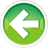# 8.2 — Floating-point and integral promotion

In lesson 4.3 -- Object sizes and the sizeof operator, we noted that C++ has minimum size guarantees for each of the fundamental types. However, the actual size of these types can vary based on the compiler and architecture.

This variability was allowed so that the `int` and `double` data types could be set to the size that maximizes performance on a given architecture. For example, a 32-bit computer will typically be able to process 32-bits of data at a time. In such cases, an `int` would likely be set to a width of 32-bits, since this is the “natural” size of the data that the CPU operates on (and likely to be the most performant).

A reminder

The number of bits a data type uses is called its width. A wider data type is one that uses more bits, and a narrower data type is one that uses less bits.

But what happens when we want our 32-bit CPU to modify an 8-bit value (such as a `char`) or a 16-bit value? Some 32-bit processors (such as the x86 series) can manipulate 8-bit or 16-bit values directly. However, doing so is often slower than manipulating 32-bit values! Other 32-bit CPUs (like the PowerPC), can only operate on 32-bit values, and additional tricks must be employed to manipulate narrower values.

Numeric promotion

Because C++ is designed to be portable and performant across a wide range of architectures, the language designers did not want to assume a given CPU would be able to efficiently manipulate values that were narrower than the natural data size for that CPU.

To help address this challenge, C++ defines a category of type conversions informally called the `numeric promotions`. A numeric promotion is the type conversion of a narrower numeric type (such as a `char`) to a wider numeric type (typically `int` or `double`) that can be processed efficiently and is less likely to have a result that overflows.

All numeric promotions are value-preserving, which means that all values in the original type are representable without loss of data or precision in the new type. Because such promotions are safe, the compiler will freely use numeric promotion as needed, and will not issue a warning when doing so.

Numeric promotion reduces redundancy

Numeric promotion solves another problem as well. Consider the case where you wanted to write a function to print a value of type `int`:

While this is straightforward, what happens if we want to also be able to print a value of type `short`, or type `char`? If type conversions did not exist, we’d have to write a different print function for `short` and another one for `char`. And don’t forget another version for `unsigned char`, `signed char`, `unsigned short`, `wchar_t`, `char8_t`, `char16_t`, and `char32_t`! You can see how this quickly becomes unmanageable.

Numeric promotion comes to the rescue here: we can write functions that have `int` and/or `double` parameters (such as the `printInt()` function above). That same code can then be called with arguments of types that can be numerically promoted to match the types of the function parameters.

Numeric promotion categories

The numeric promotion rules are divided into two subcategories: `integral promotions` and `floating point promotions`.

Floating point promotions

We’ll start with the easier one.

Using the floating point promotion rules, a value of type `float` can be converted to a value of type `double`.

This means we can write a function that takes a `double` and then call it with either a `double` or a `float` value:

In the second call to `printDouble()`, the `float` literal `4.0f` is promoted into a `double`, so that the type of argument matches the type of the function parameter.

Integral promotions

The integral promotion rules are more complicated.

Using the integral promotion rules, the following conversions can be made:

• signed char or signed short can be converted to int
• unsigned char, char8_t, and unsigned short can be converted to int if int can hold the entire range of the type, or unsigned int otherwise.
• char can be converted to int (if char is signed by default) or unsigned int (if char is unsigned by default)
• bool can be converted to int, with false becoming 0 and true becoming 1

There are a few other integral promotion rules that are used less often. These can be found at https://en.cppreference.com/w/cpp/language/implicit_conversion#Integral_promotion.

In most cases, this lets us write a function taking an `int` parameter, and then use it with a wide variety of other integral types. For example:

There are two things worth noting here. First, on some systems, some of the integral types may be converted to `unsigned int` rather than `int`. Second, some narrower unsigned types (such as `unsigned char`) will be converted to larger signed types (such as `int`). So while integral promotion is value-preserving, it is not necessarily sign-preserving.

Not all value-preserving conversions are numeric promotions

Some value-preserving type conversions (such as `int` to `long`, or `int` to `double`) are not considered to be numeric promotions in C++ (they are `numeric conversions`, which we’ll cover shortly in lesson 8.3 -- Numeric conversions).

The distinction is mostly academic. However, in certain cases, the compiler will favor numeric promotions over numeric conversions. We’ll see examples where this makes a difference when we cover overloaded function resolution (in upcoming lesson %Failed lesson reference, id XX%).8.3 -- Numeric conversionsIndex8.1 -- Implicit type conversion (coercion)